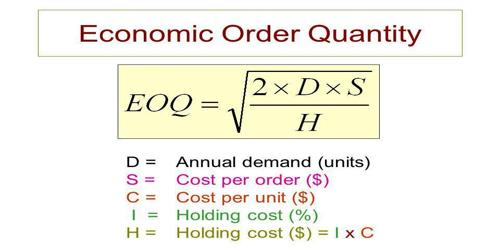# Concept behind the Economic Order Quantity (EOQ) modelThe concept behind the Economic Order Quantity (EOQ) model

Economic order quantity (EOQ) model is the method that provides the company with an order quantity. This order quantity figure is where the record holding costs and ordering costs are minimized. By using this model, the companies can minimize the costs associated with the ordering and inventory holding. In 1913, Ford W. Harris developed this formula whereas R. H. Wilson is given credit for the application and in-depth analysis on this model.

Economic order quantity (EOQ) is the order quantity of inventory that minimizes the total cost of inventory management. It is a measurement used in the field of Operations, Logistics, and Supply Management.

Definition: The economic order quantity (EOQ) is a model that is used to calculate the optimal quantity that can be purchased or produced to minimize the cost of both the carrying inventory and the processing of purchase orders or production set-ups.

Formula: Following is the formula for the economic order quantity (EOQ) model:

Q = √[(2DS)/H]

Where: Q = optimal order quantity,

D = units of annual demand,

S = cost incurred to place a single order or setup,

H = carrying a cost per unit.

This formula is derived from the following cost function:

Total cost = purchase cost + ordering cost + holding cost

Limitations of the economic order quantity model: It is necessary for the application of EOQ order that the demands remain constant throughout the year. It is also necessary that the inventory be delivered in full when the inventory levels reach zero.